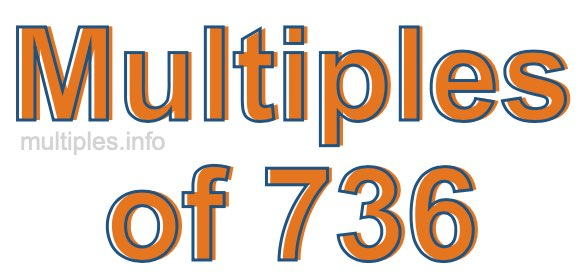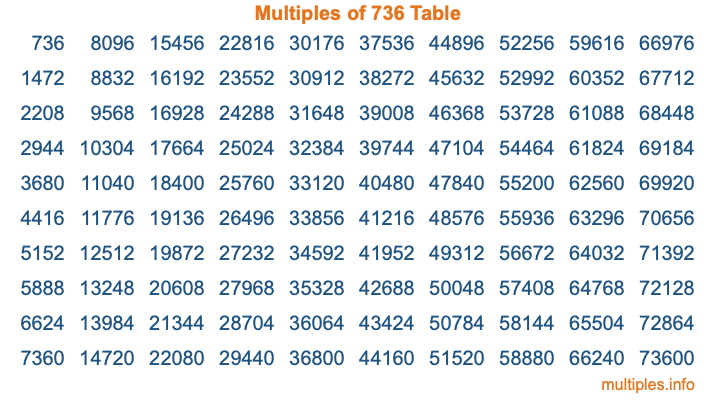Multiples of 736Welcome to the Multiples of 736 page. Here we will first teach you everything you will ever need to know about the multiples of 736, and then give you a study guide summary of everything we taught you to make sure you remember it all. Use this page to look up facts and learn information about the multiples of 736. This page will make you a multiples of seven hundred thirty-six expert!

Definition of Multiples of 736
Multiples of 736 are all the numbers that when divided by 736 equal an integer. Each of the multiples of 736 are called a multiple. A multiple of 736 is created by multiplying 736 by an integer.

Therefore, to create a list of multiples of 736, you start with 1 multiplied by 736, then 2 multiplied by 736, then 3 multiplied by 736, and so on for as long as you want. Thus, the list of the first five multiples of 736 is 736, 1472, 2208, 2944, and 3680. To see a larger list of multiples of 736, see the printable image of Multiples of 736 further down on this page. We also have a category where you can choose any nth multiple of 736.

Multiples of 736 Checker
The Multiples of 736 Checker below checks to see if any number of your choice is a multiple of 736. In other words, it checks to see if there is any number (integer) that when multiplied by 736 will equal your number. To do that, we divide your number by 736. If the the quotient is an integer, then your number is a multiple of 736.

Is  a multiple of 736?

Least Common Multiple of 736 and ...
A Least Common Multiple (LCM) is the lowest multiple that two or more numbers have in common. This is also called the smallest common multiple or lowest common multiple and is useful to know when you are adding our subtracting fractions. Enter one or more numbers below (736 is already entered) to find the LCM.

Check out our LCM Calculator if you need more details about the Least Common Multiple or if you need the LCM for different numbers for adding and subtraction fractions.

nth Multiple of 736
As we stated above, 736 is the first multiple of 736, 1472 is the second multiple of 736, 2208 is the third multiple of 736, and so on. Enter a number below to find the nth multiple of 736.

th multiple of 736

Multiples of 736 vs Factors of 736
736 is a multiple of 736 and a factor of 736, but that is where the similarities end. All postive multiples of 736 are 736 or greater than 736. All positive factors of 736 are 736 or less than 736.

Below is the beginning list of multiples of 736 and the factors of 736 so you can compare:

Multiples of 736: 736, 1472, 2208, 2944, 3680, etc.

Factors of 736: 1, 2, 4, 8, 16, 23, 32, 46, 92, 184, 368, 736

As you can see, the multiples of 736 are all the numbers that you can divide by 736 to get a whole number. The factors of 736, on the other hand, are all the whole numbers that you can multiply by another whole number to get 736.

It's also interesting to note that if a number (x) is a factor of 736, then 736 will also be a multiple of that number (x).

Multiples of 736 vs Divisors of 736
The divisors of 736 are all the integers that 736 can be divided by evenly. Below is a list of the divisors of 736.

Divisors of 736: 1, 2, 4, 8, 16, 23, 32, 46, 92, 184, 368, 736

The interesting thing to note here is that if you take any multiple of 736 and divide it by a divisor of 736, you will see that the quotient is an integer.

Multiples of 736 Table
Below is an image of the first 100 multiples of 736 in a table. The table is in chronological order, column by column. The first column has the first ten multiples of 736, the second column has the next ten multiples of 736, and so on.The Multiples of 736 Table is also referred to as the 736 Times Table or Times Table of 736. You are welcome to print out our table for your studies.

Negative Multiples of 736
Although not often discussed or needed in math, it is worth mentioning that you can make a list of negative multiples of 736 by multiplying 736 by -1, then by -2, then by -3, and so on, to get the following list of negative multiples of 736:

-736, -1472, -2208, -2944, -3680, etc.

Multiples of 736 Summary
Below is a summary of important Multiples of 736 facts that we have discussed on this page. To retain the knowledge on this page, we recommend that you read through the summary and explain to yourself or a study partner why they hold true.

There are an infinite number of multiples of 736.

A multiple of 736 divided by 736 will equal a whole number.

736 divided by a factor of 736 equals a divisor of 736.

The nth multiple of 736 is n times 736.

The largest factor of 736 is equal to the first positive multiple of 736.

736 is a multiple of every factor of 736.

736 is a multiple of 736.

A multiple of 736 divided by a divisor of 736 equals an integer.

736 divided by a divisor of 736 equals a factor of 736.

Any integer times 736 will equal a multiple of 736.

Multiples of a Number
Here you can get the multiples of another number, all with the same attention to detail as we did for multiples of 736 on this page.

Multiples of
Multiples of 737
Did you find our page about multiples of seven hundred thirty-six educational? Do you want more knowledge? Check out the multiples of the next number on our list!